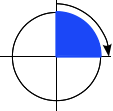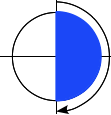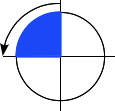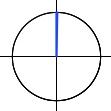# <angle>

The `<angle>` CSS data type represents an angle value expressed in degrees, gradians, radians, or turns. It is used, for example, in `rotate` and `skew` `transform` values.

Positive numbers represent clockwise angles, while negative numbers represent counterclockwise angles. For static properties of a given unit, any angle can be represented by various equivilent values. For example, `90deg` equals `-270deg`, and `1turn` equals `4turn`. For dynamic properties, like when applying a `transition` on the `transform` property, the effect will nevertheless be different.

## Syntax

An angle consists of a `<number>` followed by a unit. As with all dimensions, there is no space between the unit literal and the number. The angle unit is optional after the number `0`.

### Units

`deg`
Represents an angle in degrees. One full circle is `360deg`. Examples: `0deg`, `90deg`,` 14.23deg`.
`grad`
Represents an angle in gradians. One full circle is `400grad`. Examples: `0grad`, `100grad`,` 38.8grad`.
`rad`
Represents an angle in radians. One full circle is 2π radians which approximates to `6.2832rad`. `1rad` is 180/π degrees. Examples: `0rad`, `1.0708rad`,` 6.2832rad`.
`turn`
Represents an angle in a number of turns. One full circle is `1turn`. Examples: `0turn`, `0.25turn`,` 1.2turn`.

## ExamplesA right angle: `90deg = 100grad = 0.25turn` `≈`` 1.5708rad`A flat angle: `180deg = 200grad = 0.5turn`` ``≈`` 3.1416rad`A right angle (counterclockwise): `-90deg = -100grad = -0.25turn` `≈`` -1.5708rad`A null angle: `0 = 0deg = 0grad = 0turn = 0rad`

## Specifications

Specification Status Comment
CSS Values and Units Module Level 3
The definition of '<angle>' in that specification.
Candidate Recommendation Initial definition.

## Browser compatibility

Feature Chrome Edge Firefox (Gecko) Internet Explorer Opera Safari (WebKit)
Basic support 2 (528.17) (Yes) 3.6 (1.9.2) 9.0 (Yes) 4.0 (528.17)
`turn` unit (Yes) (Yes) 13.0 9.0 (Yes) 10
Feature Android Chrome Edge Firefox Mobile (Gecko) IE Phone Opera Mobile Safari Mobile
Basic support ? (Yes) (Yes) (Yes) ? No support ?
`turn` unit No support (Yes) (Yes) 13.0 ? No support No support

## Document Tags and Contributors

Tags:
Last updated by: mfluehr,